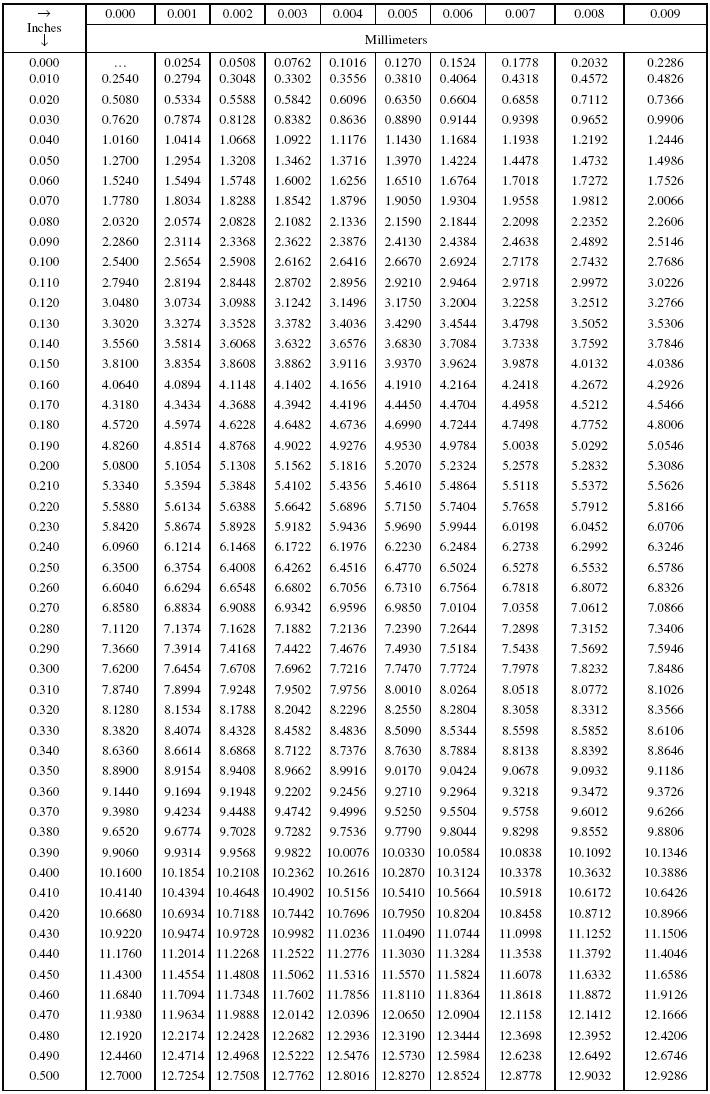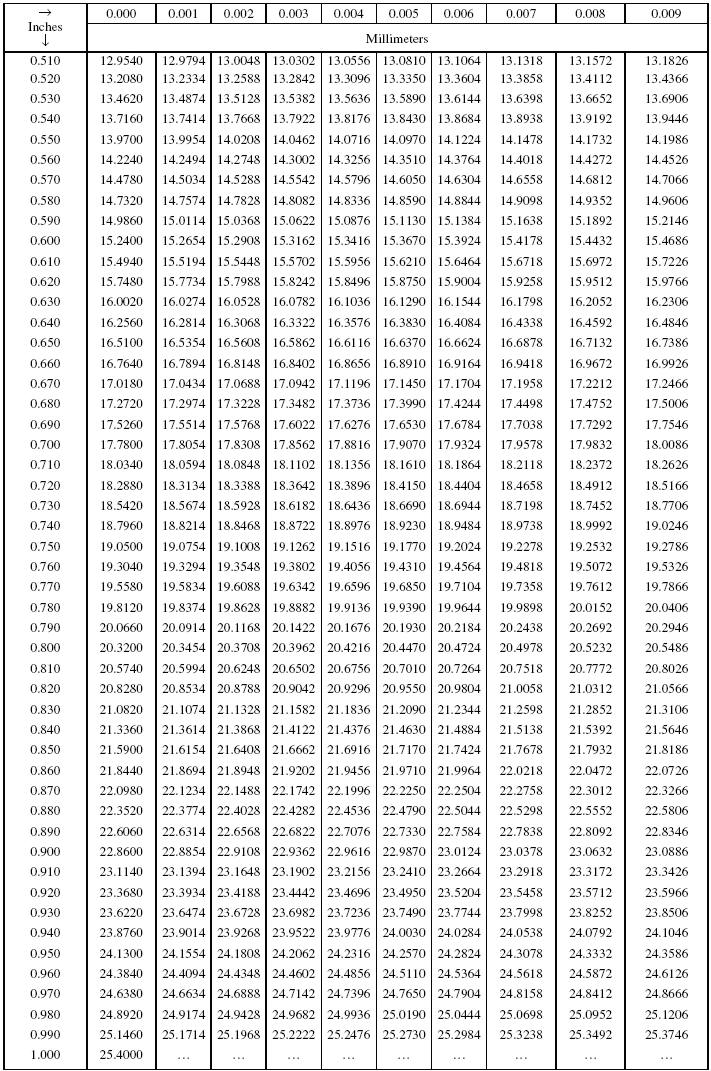Decimals of an Inch to Millimeters ConversionBased on 1 inch = 25.4 millimeters, exactly. All values in this table are exact.

Use Inch to Millimeters Conversion to obtain whole inch and other decimal equivalents to add to decimal equivalents above.

Example:

Convert 10.9983 in. to mm. Solution: 10.9983 in. = 254.0 + 25.3492 + 0.00762 = 279.35682 mm.

Recherche personnalisée